Related Articles

# Title alignment in Plotly

• Last Updated : 29 Jul, 2021

In this article, we are going to discuss title assignment and alignment using plotly module.

In order to align titles in visualization using plotly module, we are going to use the update_layout() method.

Syntax:

plotly.graph_objects.Figure.update_layout(title_text, title_x, title_y)

Parameters:

• title: Accepts string value as the title of the visualization.
• title_x: This parameter is used to align the title in a horizontal motion and accepts a value from 0 to 1.
• title_y: This parameter is used to align the title in a vertical motion and accepts a value from 0 to 1.

Let’s implement the method on the below examples:

Example 1

## Python3

 `# import all required libraries` `import` `numpy as np` `import` `plotly` `import` `plotly.graph_objects as go` `import` `plotly.offline as pyo` `from` `plotly.offline ``import` `init_notebook_mode`   `init_notebook_mode(connected``=``True``)`   `# generating 150 random integers` `# from 1 to 50` `x ``=` `np.random.randint(low``=``1``, high``=``50``, size``=``150``)``*``0.1`   `# generating 150 random integers` `# from 1 to 50` `y ``=` `np.random.randint(low``=``1``, high``=``50``, size``=``150``)``*``0.1`   `# plotting scatter plot` `fig ``=` `go.Figure(data``=``go.Scatter(x``=``x, y``=``y, mode``=``'markers'``))`   `# title alignment` `fig.update_layout(title_text``=``'Dots'``)`   `fig.show()`

Output: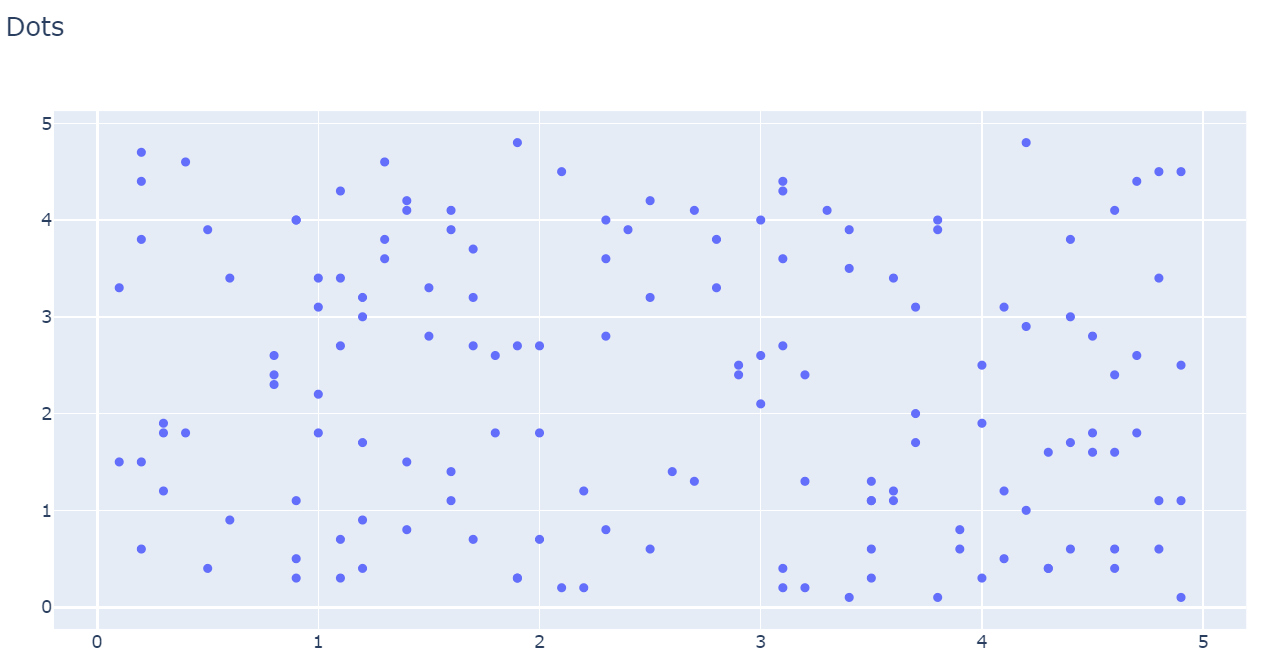Example 2

## Python3

 `# import all required libraries ` `import` `numpy as np ` `import` `plotly ` `import` `plotly.graph_objects as go ` `import` `plotly.offline as pyo ` `from` `plotly.offline ``import` `init_notebook_mode `   `init_notebook_mode(connected ``=` `True``) `   `# different individual parts in ` `# total chart ` `countries``=``[``'India'``, ``'canada'``, ` `        ``'Australia'``,``'Brazil'``, ` `        ``'Mexico'``,``'Russia'``, ` `        ``'Germany'``,``'Switzerland'``, ` `        ``'Texas'``] `   `# values corresponding to each ` `# individual country present in ` `# countries ` `values ``=` `[``4500``, ``2500``, ``1053``, ``500``, ` `        ``3200``, ``1500``, ``1253``, ``600``, ``3500``] `   `# plotting pie chart ` `fig ``=` `go.Figure(data``=``[go.Pie(labels``=``countries, ` `                    ``values``=``values)]) `   `# title alignment` `fig.update_layout(title_text``=``'Pie'``,title_y``=``0.5``)`   `fig.show()`

Output:

With title_y=0.5, the title should be in center.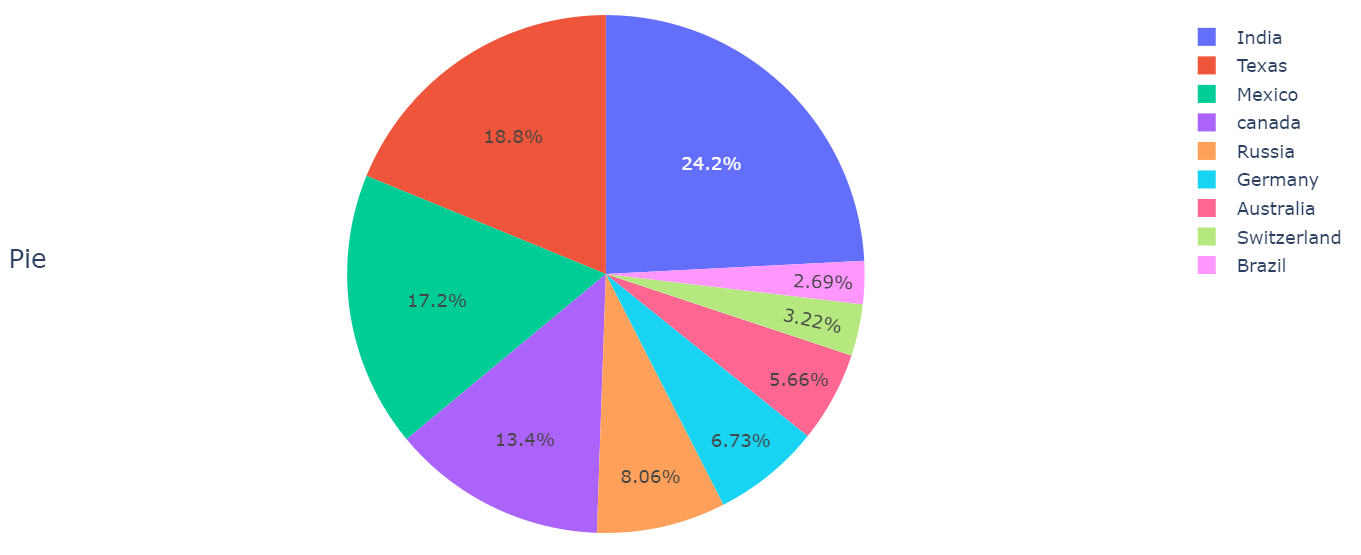When title_y = 0.1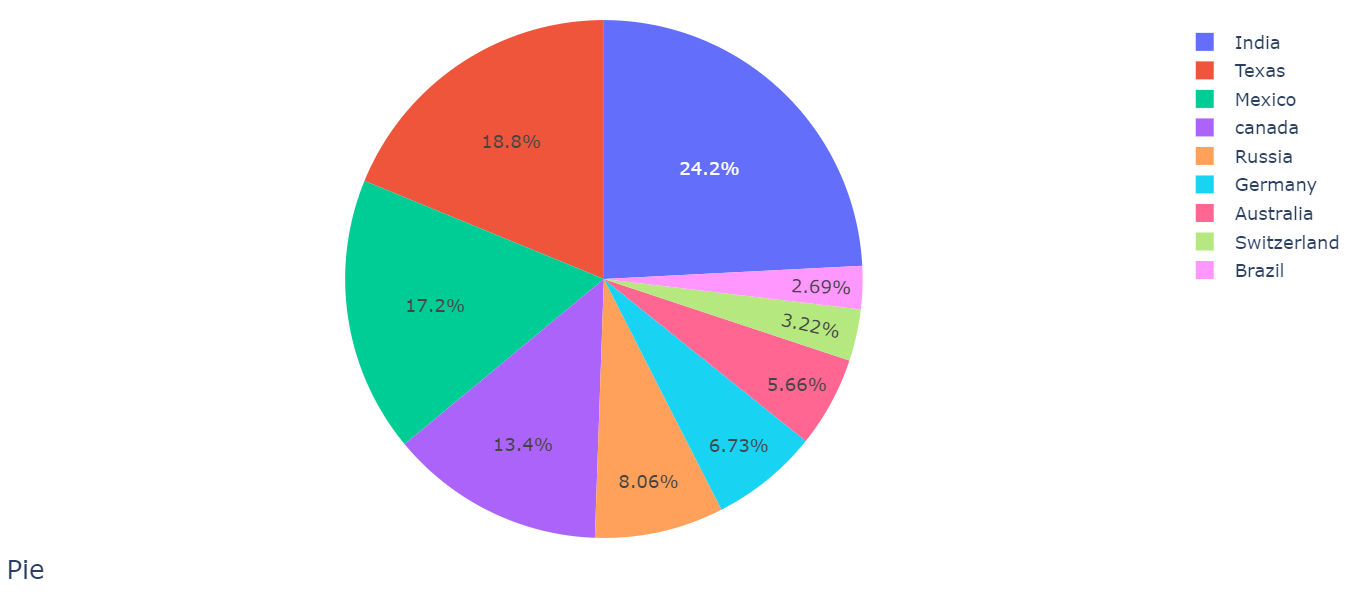When title_y = 1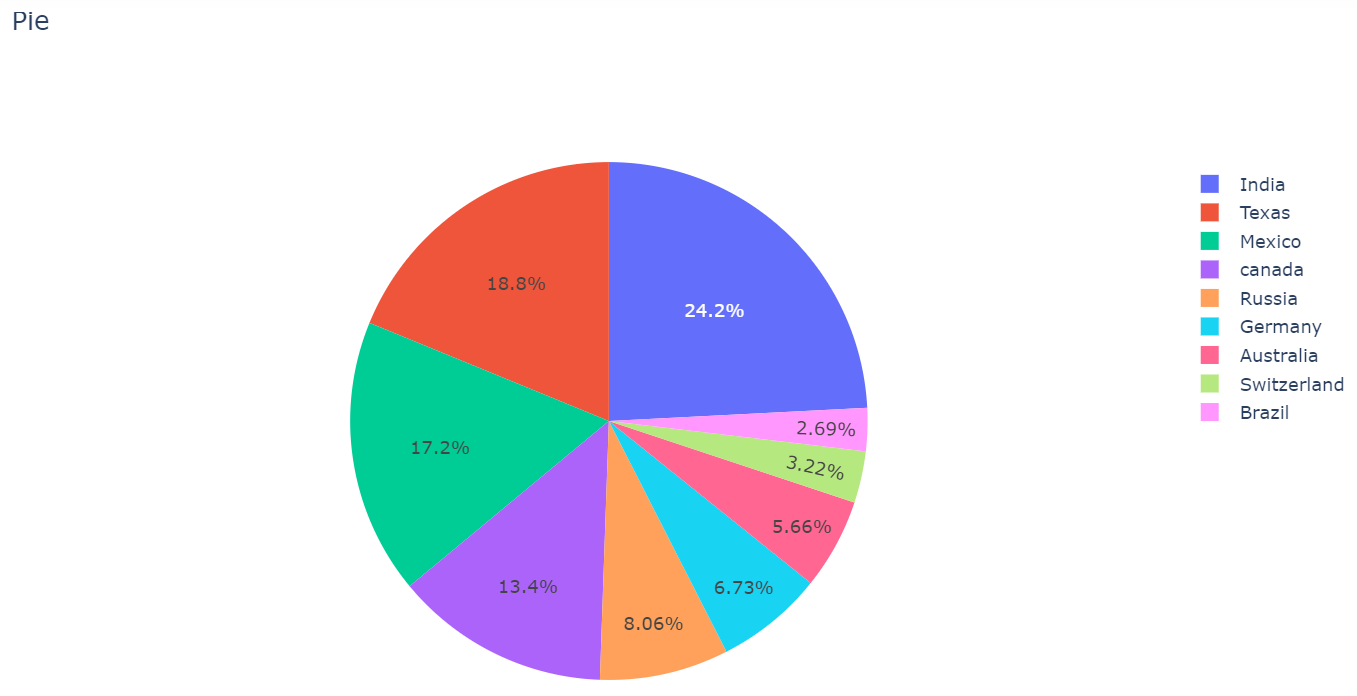Example 3

## Python3

 `# import required libraries` `import` `dash` `import` `dash_core_components as dcc` `import` `dash_html_components as html` `import` `plotly.express as px` `import` `pandas as pd`   `external_stylesheets ``=` `[``'https://codepen.io/chriddyp/pen/bWLwgP.css'``]`   `app ``=` `dash.Dash(__name__, external_stylesheets``=``external_stylesheets)`   `# assume you have a "long-form" data frame` `df ``=` `pd.DataFrame({` `    ``"Year"``: [``"2015"``, ``"2016"``, ``"2017"``, ``"2015"``, ``"2016"``, ``"2017"``],` `    ``"Revenue"``: [``4``, ``1``, ``2``, ``2``, ``4``, ``5``],` `    ``"Company"``: [``"ABC Pvt. Ltd."``, ``"ABC Pvt. Ltd."``, ``"ABC Pvt. Ltd."``, ``"XYZ Pvt. Ltd."``, ``"XYZ Pvt. Ltd."``, ``"XYZ Pvt. Ltd."``]` `})`   `# depict visualization` `fig ``=` `px.bar(df, x``=``"Year"``, y``=``"Revenue"``, color``=``"Company"``, barmode``=``"group"``)`   `app.layout ``=` `html.Div(children``=``[`   `    ``dcc.Graph(` `        ``id``=``'example-graph'``,` `        ``figure``=``fig` `    ``)` `])`   `# allign title` `fig.update_layout(title_text``=``'Revenue'``, title_x``=``0.5``)`   `if` `__name__ ``=``=` `'__main__'``:` `    ``app.run_server(debug``=``True``)`

Output:

With title_x=0.5, the title should be in center.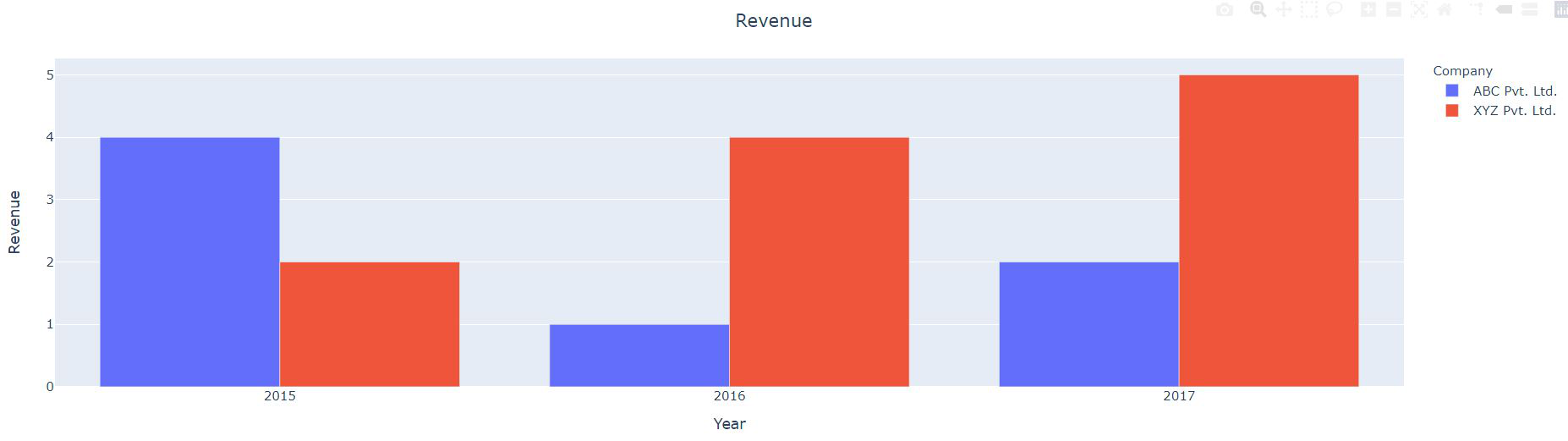When title_x = 0.3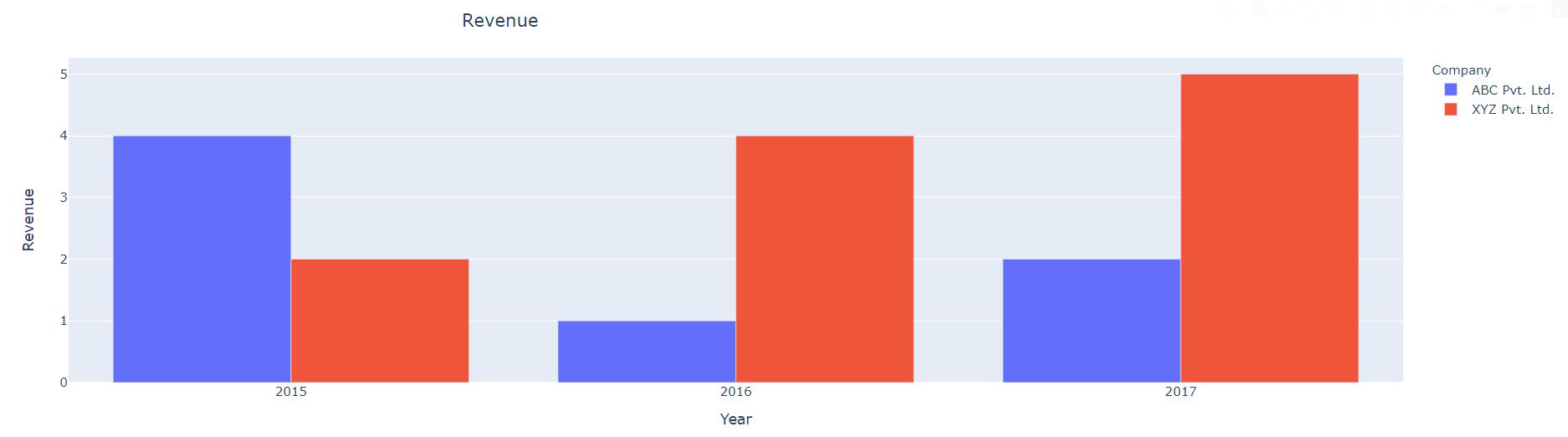When title_x = 1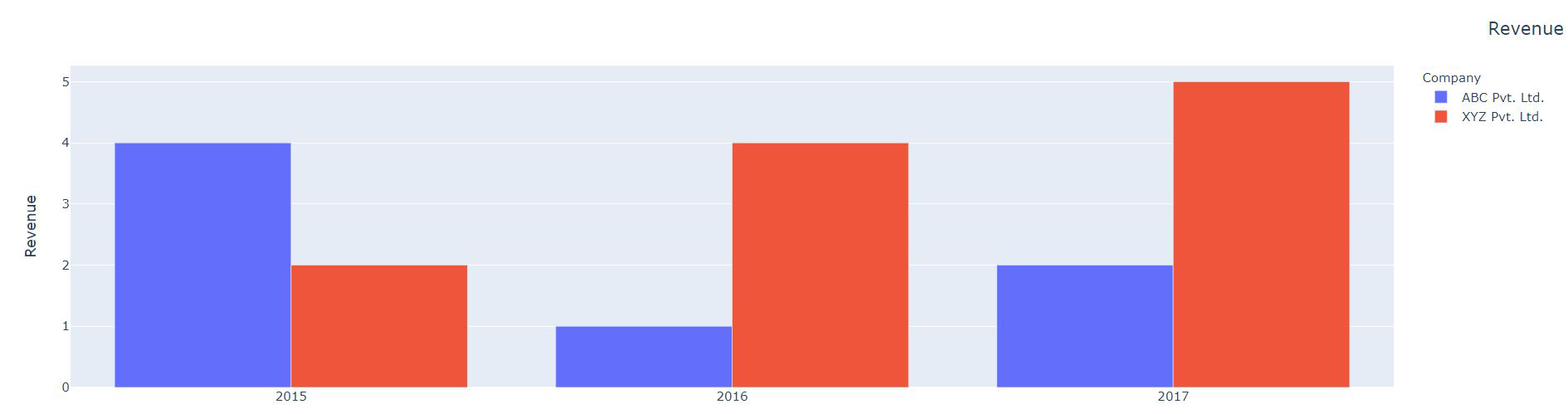Attention geek! Strengthen your foundations with the Python Programming Foundation Course and learn the basics.

To begin with, your interview preparations Enhance your Data Structures concepts with the Python DS Course. And to begin with your Machine Learning Journey, join the Machine Learning – Basic Level Course

My Personal Notes arrow_drop_up
Recommended Articles
Page :